Test: Basic Laws- 2

# Test: Basic Laws- 2

Test Description

## 15 Questions MCQ Test Topicwise Question Bank for Electrical Engineering | Test: Basic Laws- 2

Test: Basic Laws- 2 for Electrical Engineering (EE) 2023 is part of Topicwise Question Bank for Electrical Engineering preparation. The Test: Basic Laws- 2 questions and answers have been prepared according to the Electrical Engineering (EE) exam syllabus.The Test: Basic Laws- 2 MCQs are made for Electrical Engineering (EE) 2023 Exam. Find important definitions, questions, notes, meanings, examples, exercises, MCQs and online tests for Test: Basic Laws- 2 below.
Solutions of Test: Basic Laws- 2 questions in English are available as part of our Topicwise Question Bank for Electrical Engineering for Electrical Engineering (EE) & Test: Basic Laws- 2 solutions in Hindi for Topicwise Question Bank for Electrical Engineering course. Download more important topics, notes, lectures and mock test series for Electrical Engineering (EE) Exam by signing up for free. Attempt Test: Basic Laws- 2 | 15 questions in 45 minutes | Mock test for Electrical Engineering (EE) preparation | Free important questions MCQ to study Topicwise Question Bank for Electrical Engineering for Electrical Engineering (EE) Exam | Download free PDF with solutions
 1 Crore+ students have signed up on EduRev. Have you?
Test: Basic Laws- 2 - Question 1

### The current I in the circuit shown below is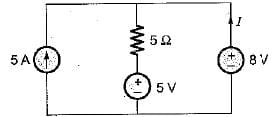Detailed Solution for Test: Basic Laws- 2 - Question 1

Let the current flowing through 5 Ω resistor be I' Applying KCL, we get
5 + I = I'.
Applying KVL, we get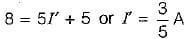So,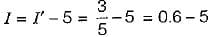= -4.4 A

Test: Basic Laws- 2 - Question 2

### For the circuit shown below, match List - I with List-II and select the correct answer using the codes given below the lists: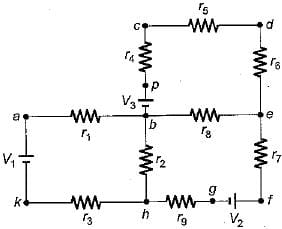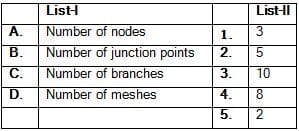Codes: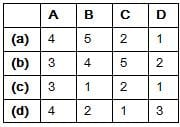Detailed Solution for Test: Basic Laws- 2 - Question 2

Nodes = 10 (a, b, c, d, e, f, g, h, k, p)
Junction points = 3(b, e, h)

Test: Basic Laws- 2 - Question 3

### The value of current I in the circuit shown below for V = 2 volt is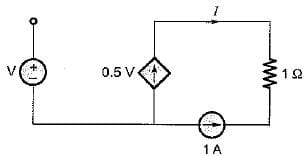Detailed Solution for Test: Basic Laws- 2 - Question 3

Applying KCL at the node connecting 0.5 V current source and 1 A current source, we get
1 + I = 0.5 V
For v - 2 volt, 1 + I = 1
or, I = 0 A

Test: Basic Laws- 2 - Question 4

For the circuit shown below, the value of (V1+ V2) is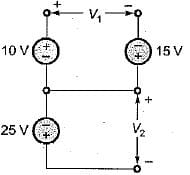Detailed Solution for Test: Basic Laws- 2 - Question 4

Using KVL in the loop, we have
V= 10 + 15 = 25 volts
Also, V2 = -25 volts
∴ V1 + V2 = 25 - 25 = 0 volts

Test: Basic Laws- 2 - Question 5

The value of voltage source to be connected across the terminals X and Y so that drop across the 10 Ω resistor is 45 V is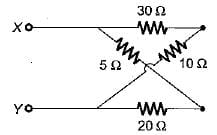Detailed Solution for Test: Basic Laws- 2 - Question 5

Let the required voltage be V
Then, voltage across 10 Ω resistor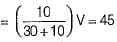or,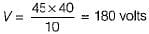Hence, required voltage is
V = 180 volts

Test: Basic Laws- 2 - Question 6

In a voltage divider circuit as shown below with two resistances R1 and R2, the voltage drop V1 is twice the drop V2 across R2. The value of R1, and R2 will be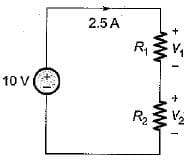Detailed Solution for Test: Basic Laws- 2 - Question 6

Using Ohm’s law,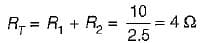Given, V1 = 2V2
As I is same therefore,
R1 = 2R2
∴ RT = R1 + R2 = 2R2 + R2 = 3R2
So, RT = 3R2 = 4Ω
or,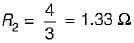and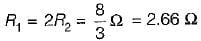Test: Basic Laws- 2 - Question 7

A delta connected load is shown in figure below. The equivalent star connection has a value of R in Ω is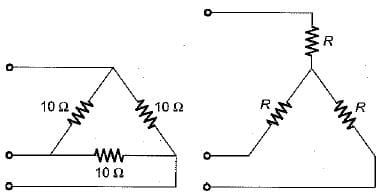Detailed Solution for Test: Basic Laws- 2 - Question 7

We know that
RΔ = 3 RY
or,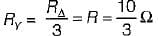Test: Basic Laws- 2 - Question 8

The voltage drop across the 2 Ω resistor for the circuit shown below is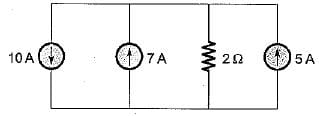Detailed Solution for Test: Basic Laws- 2 - Question 8

On combining the current sources, the circuit is reduced as shown below.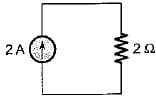Hence, voltage drop across the 2Ω resistor
= 2 x 2 = 4 volts

Test: Basic Laws- 2 - Question 9

For the circuit shown below, the sum of the unknown currents I1, I2, l3 and I4 is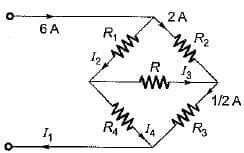Detailed Solution for Test: Basic Laws- 2 - Question 9

Using KCL,
I2 = 6 - 2 = 4 A
Also,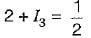or,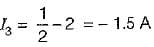and I4 = I- I3 = 4 - (-1.5) = 5.5 A
Also, I1 = 6 A
∴ I1 + I2 + I3 + I4 = 6 + 4 - 1.5 + 5.5
= 15.5 - 1.5 = 14A

Test: Basic Laws- 2 - Question 10

In the delta equivalent of the given star connected circuit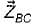is equal to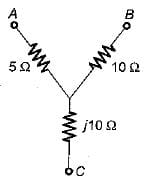Detailed Solution for Test: Basic Laws- 2 - Question 10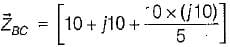= (10 + j10 + j20)
= (10 + j30) Ω

Test: Basic Laws- 2 - Question 11

Four resistances 80 Ω, 50 Ω, 25 Ω and R are connected in parallel. Current through 25 Ω resistance is 4 A. The total current of the supply is 10 A. The value of R will be

Detailed Solution for Test: Basic Laws- 2 - Question 11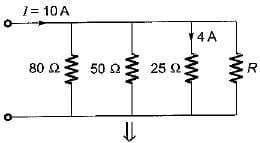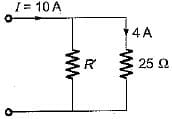Given, I25Ω=4 A
Here, R' = 80║50║R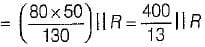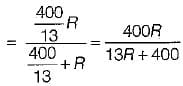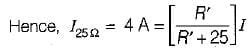or,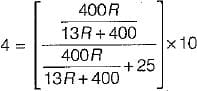or,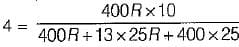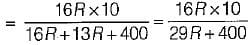or, 29R + 400 = 40R or 11R = 400
or, R = 36.36 Ω

Test: Basic Laws- 2 - Question 12

For the circuit shown below, the equivalent resistance will be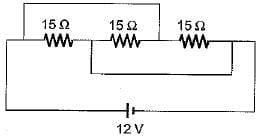Detailed Solution for Test: Basic Laws- 2 - Question 12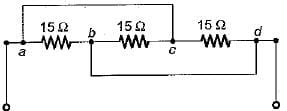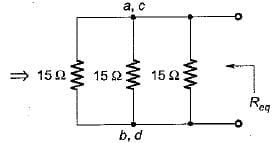Here, a and c are at equipotential.
Also, b and d are at equipotential
∴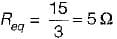Test: Basic Laws- 2 - Question 13

Three resistors of R Ω each are connected to form a triangle. The resistance between any two terminals will be

Detailed Solution for Test: Basic Laws- 2 - Question 13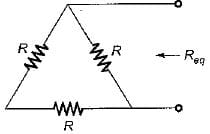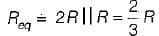Test: Basic Laws- 2 - Question 14

A network has 10 nodes and 17 branches. The number of different node pair voltage would be

Detailed Solution for Test: Basic Laws- 2 - Question 14

Number of different node-pair voltage = Number of KCL equations = n - 1 =10 - 1 = 9

Test: Basic Laws- 2 - Question 15

Kirchhoff's laws are not applicable to circuits with

## Topicwise Question Bank for Electrical Engineering

207 tests
Information about Test: Basic Laws- 2 Page
In this test you can find the Exam questions for Test: Basic Laws- 2 solved & explained in the simplest way possible. Besides giving Questions and answers for Test: Basic Laws- 2, EduRev gives you an ample number of Online tests for practice

## Topicwise Question Bank for Electrical Engineering

207 tests(Scan QR code)